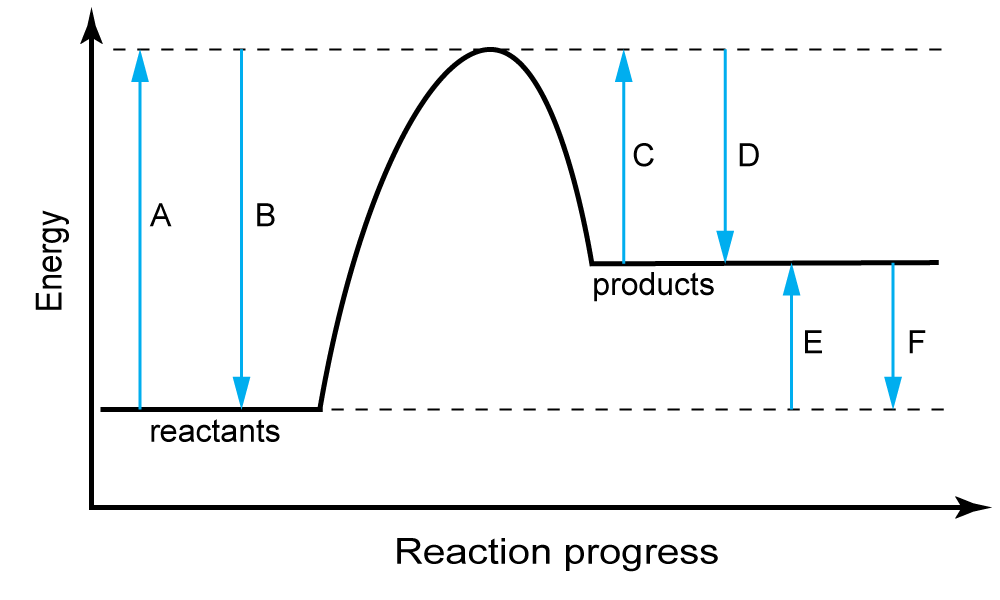Chemistry µGCSE:

Energy Changes

10 quick questions - for GCSE and iGCSE

10 minutes maximum! (can you do it in 5?)

1. Chemical reactions in which heat energy is given out are described as …..
• A. ..exothermic and produce a temperature rise in solution
• B. ..exothermic and produce a temperature drop in solution
• C. …endothermic and produce a temperature rise in solution
• D. ..endothermic and produce a temperature drop in solution

2. The table below gives information about the temperature changes in 3 reactions:

 Reaction Initial temperature / oC Final temperature / oC I 25.6 32.4 II 22.1 19.3 III 21.5 48.8

The reactions which are exothermic are:

• A.  I and II
• B.   I and III
• C.   II only
• D.   I, II and III
3. Which of the following types of chemical reactions are always endothermic ?
• A.  Combustion
• B.  Neutralisation
• C.  Displacement
• D. Thermal Decomposition

Q4-6 refer to the following reaction profile:4. This diagram represents the reaction profile for an …
• A.  exothermic reaction as the products are at a lower energy level than the reactants.
• B. exothermic reaction as the products are at a higher energy level than the reactants.
• C. endothermic reaction as the products are at a lower energy level than the reactants.
• D. endothermic reaction as the products are at a higher energy level than the reactants.
5. The enthalpy change (energy change) for this reaction is correctly labelled by line .
6. Line D represents ….
• A.   the enthalpy (energy) change for the reaction.
• B.   the energy needed to break the bonds in the reactants.
• C.   the energy released on forming bonds in products.
• D.   the activation energy for the reaction.

Q7 – 10.   Water at 25 °C was used to dissolve ammonium nitrate (NH4NO3).

The ammonium nitrate disappeared and a temperature of 21°C was recorded immediately afterwards.

The student used a polystyrene cup to carry out this experiment as shown here.7. The reason for using a polystyrene cup rather than a beaker is that a polystyrene cup is…..

• A.   ….not breakable
• B.   …. cheaper than a beaker
• C.   ….a good insulator of heat
• D.   ….a good conductor of heat

8. The student correctly concluded that the dissolving of ammonium nitrate was …

• A. exothermic because the temperature decreased
• B. endothermic because the temperature decreased
• C. exothermic because the ammonium nitrate disappeared
• D. endothermic because the ammonium nitrate disappeared

9. Which of the following energy level diagrams correctly represents this reaction?10. Estimate the temperature of the solution if the volume of water was halved but the same mass of ammonium nitrate used.

• A.    17°C
• B.    21°C
• C.    23°C
• D.    25°C

Image by Republica from Pixabay